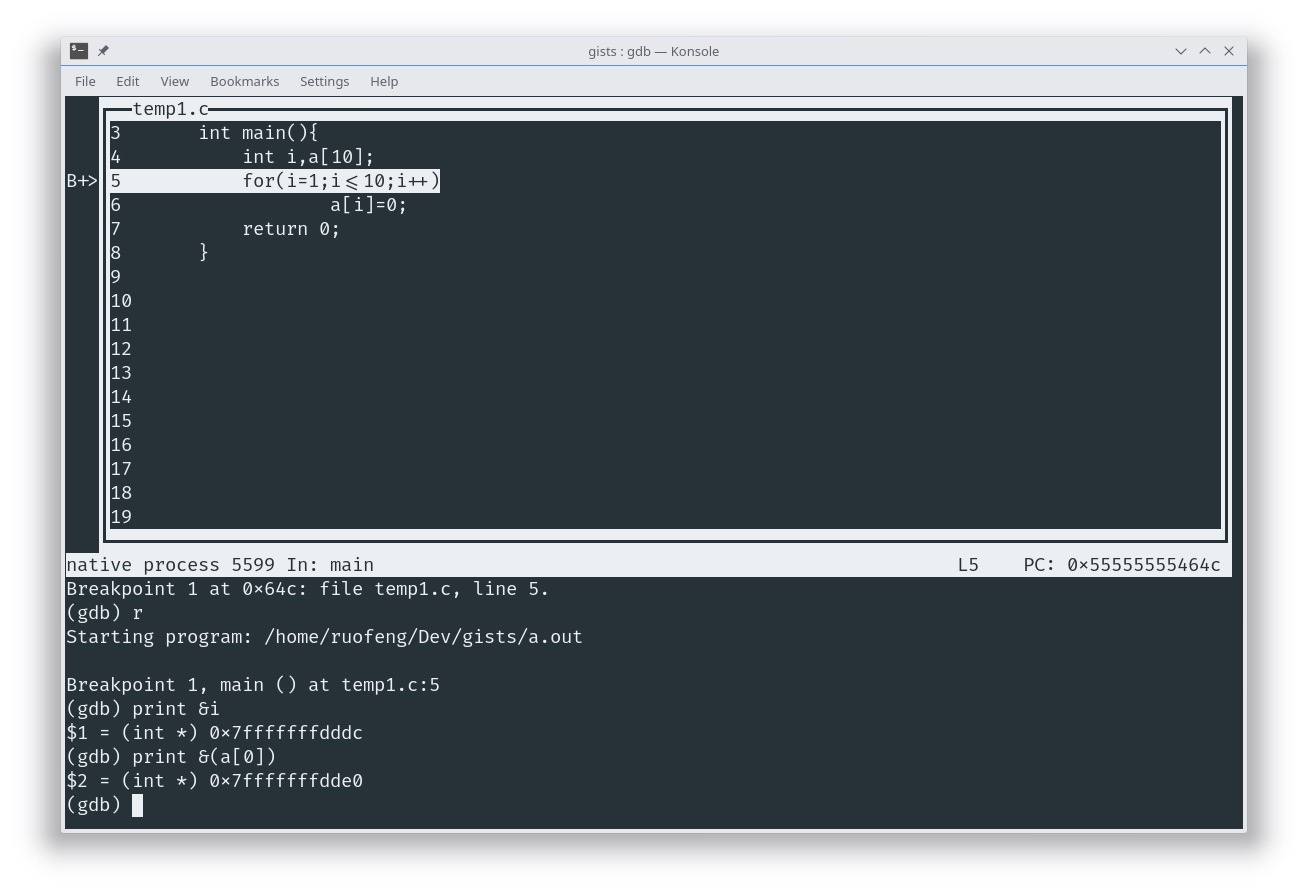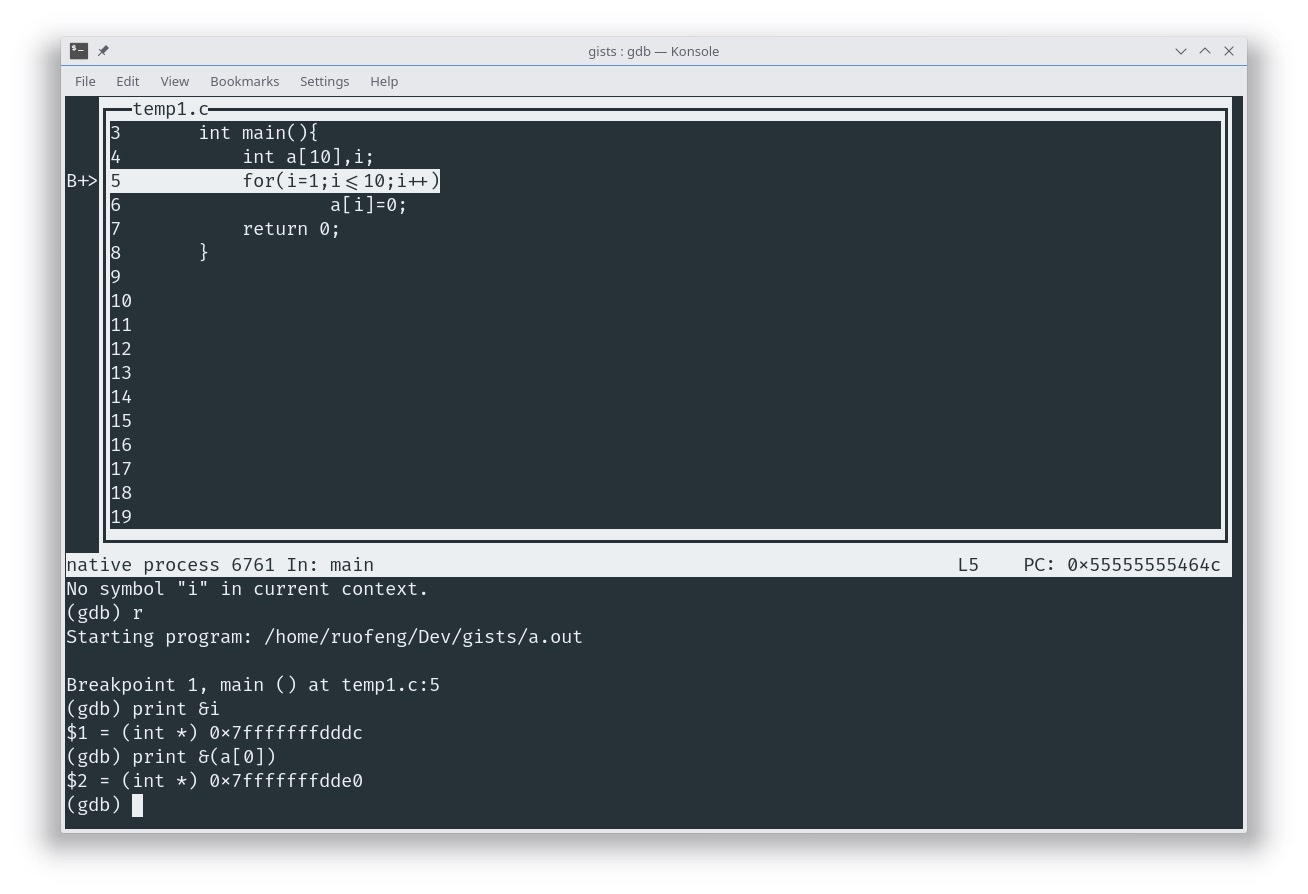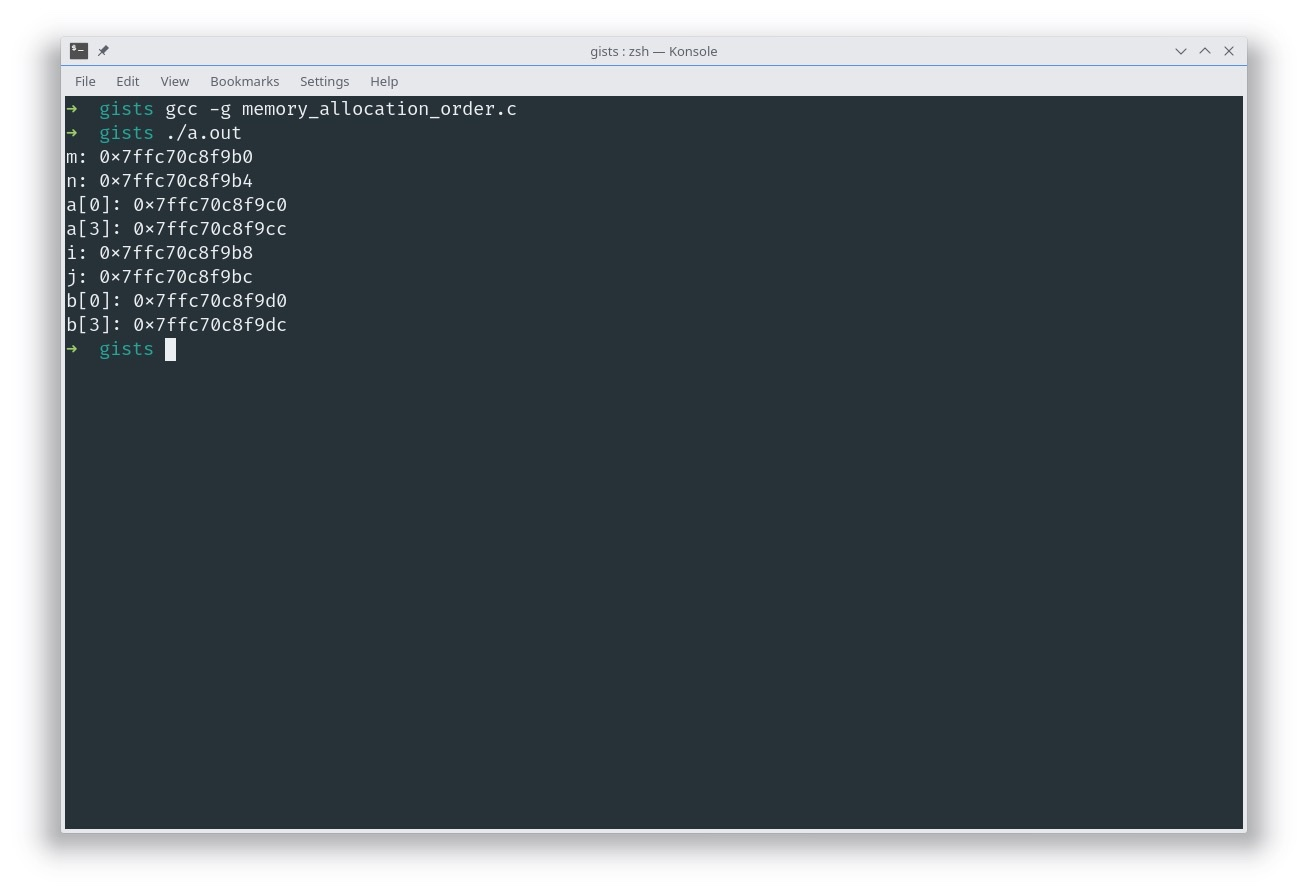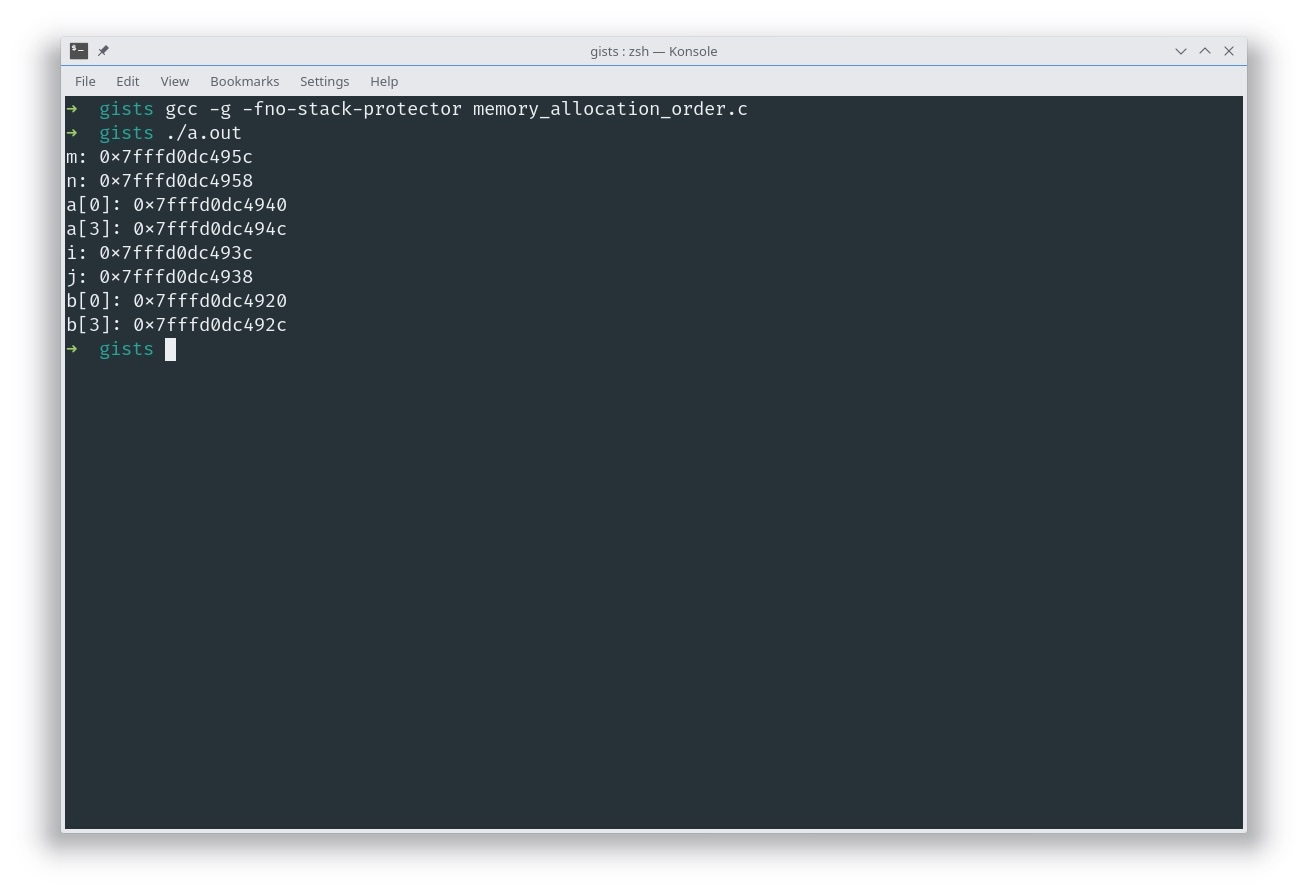C 语言中变量可能并不是按照代码中声明变量的顺序分配在内存中的。

## 问题的发生

``````int i, a;
for (i = 1; i <= 10; i++)
a[i] = 0;
````````````int a, i;
for (i = 1; i <= 10; i++)
a[i] = 0;
``````

i 的地址依然在 a 前面## 再次尝试

``````int main(){
int m=0;
int n=0;
int a={1,2,3,4};
int i=0;
int j=0;
int b={5,6,7,8};
printf("m: %p\n",&m);
printf("n: %p\n",&n);
printf("a: %p\n",&(a));
printf("a: %p\n",&(a));
printf("i: %p\n",&i);
printf("j: %p\n",&j);
printf("b: %p\n",&(b));
printf("b: %p\n",&(b));
return 0;
``````## 问题所在：GCC 的栈溢出保护